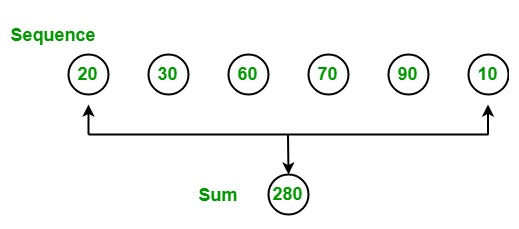# LINQ | How to find the sum of the given Sequence?

In LINQ, you can find the sum of the given numeric elements by using the Sum() method. This method calculates the sum of the numeric value present in the given sequence. It does not support query syntax in C#, but it supports in VB.NET. It is available in both Enumerable and Queryable classes in C#. It can work with nullable, non-nullable decimal, double, floats, int, etc. values.Example 1:

 `// C# program to illustrate how to ` `// find the sum of the given sequence ` `using` `System; ` `using` `System.Linq; ` ` `  `class` `GFG { ` ` `  `    ``// Main Method ` `    ``static` `public` `void` `Main() ` `    ``{ ` ` `  `        ``int``[] sequence = { 20, 30, 50, 78, 90, ` `                           ``79, 89, 99, 97, 29 }; ` ` `  `        ``// Display the sequence ` `        ``Console.WriteLine(``"The Sequence is: "``); ` ` `  `        ``foreach``(``int` `s ``in` `sequence) ` `        ``{ ` `            ``Console.WriteLine(s); ` `        ``} ` ` `  `        ``// Finding sum of the given sequence ` `        ``// Using Sum function ` `        ``int` `result = sequence.Sum(); ` `        ``Console.WriteLine(``"The sum of the given "` `                              ``+ ``"sequence is: {0}"``, ` `                          ``result); ` `    ``} ` `} `

Output:

```The Sequence is:
20
30
50
78
90
79
89
99
97
29
The sum of the given sequence is: 661
```

Example 2:

 `// C# program to find the sum ` `// of the salary of the employee ` `using` `System; ` `using` `System.Linq; ` `using` `System.Collections.Generic; ` ` `  `// Employee details ` `public` `class` `employee { ` `    ``public` `int` `emp_id ` `    ``{ ` `        ``get``; ` `        ``set``; ` `    ``} ` `    ``public` `string` `emp_name ` `    ``{ ` `        ``get``; ` `        ``set``; ` `    ``} ` `    ``public` `string` `emp_gender ` `    ``{ ` `        ``get``; ` `        ``set``; ` `    ``} ` `    ``public` `string` `emp_hire_date ` `    ``{ ` `        ``get``; ` `        ``set``; ` `    ``} ` `    ``public` `int` `emp_salary ` `    ``{ ` `        ``get``; ` `        ``set``; ` `    ``} ` `} ` ` `  `class` `GFG { ` ` `  `    ``// Main method ` `    ``static` `public` `void` `Main() ` `    ``{ ` `        ``List emp = ``new` `List() { ` `            ``new` `employee() { emp_id = 209, emp_name = ``"Anjita"``, emp_gender = ``"Female"``, emp_hire_date = ``"12/3/2017"``, emp_salary = 20000 }, ` `                ``new` `employee() { emp_id = 210, emp_name = ``"Soniya"``, emp_gender = ``"Female"``, emp_hire_date = ``"22/4/2018"``, emp_salary = 30000 }, ` `                ``new` `employee() { emp_id = 211, emp_name = ``"Rohit"``, emp_gender = ``"Male"``, emp_hire_date = ``"3/5/2016"``, emp_salary = 40000 }, ` `                ``new` `employee() { emp_id = 212, emp_name = ``"Supriya"``, emp_gender = ``"Female"``, emp_hire_date = ``"4/8/2017"``, emp_salary = 40000 }, ` `                ``new` `employee() { emp_id = 213, emp_name = ``"Anil"``, emp_gender = ``"Male"``, emp_hire_date = ``"12/1/2016"``, emp_salary = 40000 }, ` `                ``new` `employee() { emp_id = 214, emp_name = ``"Anju"``, emp_gender = ``"Female"``, emp_hire_date = ``"17/6/2015"``, emp_salary = 50000 }, ` `        ``}; ` ` `  `        ``// Find the sum of the salary ` `        ``// Using Sum () method ` `        ``var` `res = emp.Sum(a = > a.emp_salary); ` `        ``Console.WriteLine(``"Total salary of the Employees: {0}"``, res); ` `    ``} ` `} `

Output:

```Total salary of the Employees: 220000
```

My Personal Notes arrow_drop_upCheck out this Author's contributed articles.

If you like GeeksforGeeks and would like to contribute, you can also write an article using contribute.geeksforgeeks.org or mail your article to contribute@geeksforgeeks.org. See your article appearing on the GeeksforGeeks main page and help other Geeks.

Please Improve this article if you find anything incorrect by clicking on the "Improve Article" button below.

Article Tags :

Be the First to upvote.

Please write to us at contribute@geeksforgeeks.org to report any issue with the above content.